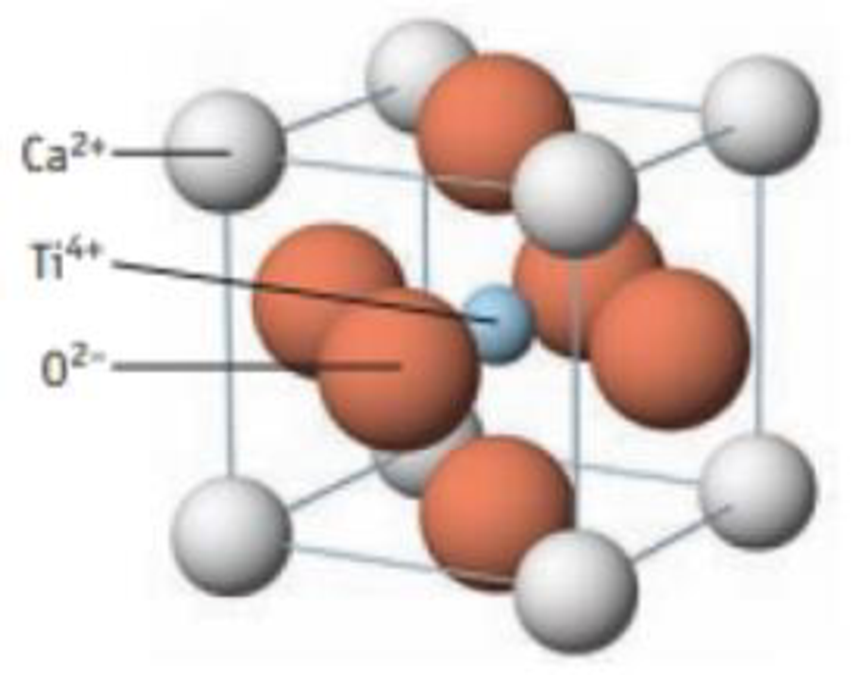Chapter 12, Problem 5PS

Chapter
Section
Textbook Problem

One way of viewing the unit cell of perovskite was illustrated in Example 12.2. Another way is shown here. Prove that this view also leads to a formula of CaTiO3.Perovskite unit cell

Interpretation Introduction

Interpretation:

From the view, the formula CaTiO3 has to be proved.

Explanation

The Ca2+ ion at eight corners therefore,

Number of Ca2+atoms per unit=(8corneratoms×1/8)Number of Ca2+atoms per unit=1

The O2- ion at six faces therefore,

Number of O2-atoms per unit=(6faceO2-

Still sussing out bartleby?

Check out a sample textbook solution.

See a sample solution

The Solution to Your Study Problems

Bartleby provides explanations to thousands of textbook problems written by our experts, many with advanced degrees!

Get Started